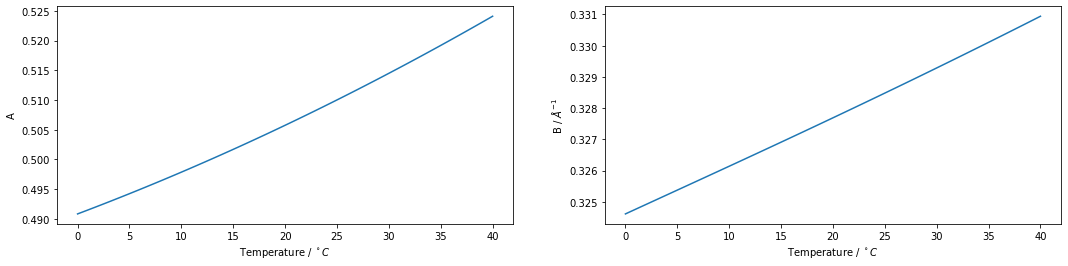Debye-Hückel theory¶

The Debye-Hückel equation describes the non-ideality of dilute electrolytes. In this notebook we will showcase how ChemPy can work both with floating points representations (and then optionally with units) and with symbolic input.

In :
from math import log as ln

import numpy as np
import matplotlib.pyplot as plt

from chempy.units import default_constants as consts, default_units as u
from chempy.properties.water_density_tanaka_2001 import water_density
from chempy.properties.water_permittivity_bradley_pitzer_1979 import water_permittivity
import chempy.electrolytes as dh
import chempy.symbolic
In :
def DH_A_B(T):
eps_r = water_permittivity(T, 1*u.bar, units=u)
rho = water_density(T, units=u)
b0 = 1 * u.mol/u.kg
A = dh.A(eps_r, T, rho, b0=b0, constants=consts, units=u).simplified/ln(10)
B = dh.B(eps_r, T, rho, b0=b0, constants=consts, units=u).rescale(1/u.angstrom)
return A, B
In :
DH_A_B(298.15*u.kelvin)
Out:
(array(0.51001004) * dimensionless, array(0.32848104) * 1/angstrom)
In :
T = (np.linspace(0, 40)+273.15)*u.Kelvin
A, B = DH_A_B(T)
%matplotlib inline
plt.figure(figsize=(18,4))
plt.subplot(1, 2, 1)
plt.plot(T-273.15*u.K, A)
plt.xlabel(r"Temperature / $^\circ C$")
plt.ylabel("A")
plt.subplot(1, 2, 2)
plt.plot(T-273.15*u.K, B)
plt.xlabel(r"Temperature / $^\circ C$")
plt.ylabel("B / $\AA^{-1}$")
Out:
Text(0, 0.5, 'B / $\\AA^{-1}$')In :
import sympy as sp
sp.init_printing()
In :
constants = chempy.symbolic.get_constant_symbols()
Out:
$\displaystyle N_{A}$
In :
var_symbs = A, B, eps_r, T, rho, b0, I, z, a, I0, C, gamma = sp.symbols('A B epsilon_r T rho b^o I z a I^o C gamma')
one = sp.S(1)
var_symbs
Out:
$\displaystyle \left( A, \ B, \ \epsilon_{r}, \ T, \ \rho, \ b^{o}, \ I, \ z, \ a, \ I^{o}, \ C, \ \gamma\right)$
In :
A_expr = dh.A(eps_r, T, rho, b0, constants, backend='sympy')
sp.Eq(A, A_expr)
Out:
$\displaystyle A = \frac{\sqrt{2} F^{3} \sqrt{\frac{b^{o} \rho}{N_{A}^{3} T^{3} \epsilon_{0}^{3} \epsilon_{r}^{3} k_{B}^{3}}}}{8 N_{A} \pi}$
In :
B_expr = dh.B(eps_r, T, rho, b0, constants, backend=sp)
sp.Eq(B, B_expr)
Out:
$\displaystyle B = \sqrt{2} F \sqrt{\frac{b^{o} \rho}{R T \epsilon_{0} \epsilon_{r}}}$
In :
sp.Eq(sp.log(gamma), dh.limiting_log_gamma(I, z, A, I0=I0, backend=sp))
Out:
$\displaystyle \log{\left(\gamma \right)} = - A z^{2} \sqrt{\frac{I}{I^{o}}}$
In :
sp.Eq(sp.log(gamma), dh.extended_log_gamma(I, z, a, A, B, C=C, I0=I0, backend=sp))
Out:
$\displaystyle \log{\left(\gamma \right)} = - \frac{A z^{2} \sqrt{\frac{I}{I^{o}}}}{B a \sqrt{\frac{I}{I^{o}}} + 1} + \frac{C I}{I^{o}}$

One motivation for having these expressions available as functions in ChemPy is that the more equations we take from (well tested) libraries the smaller is the risk of introducing typos (which can take a long time to spot).